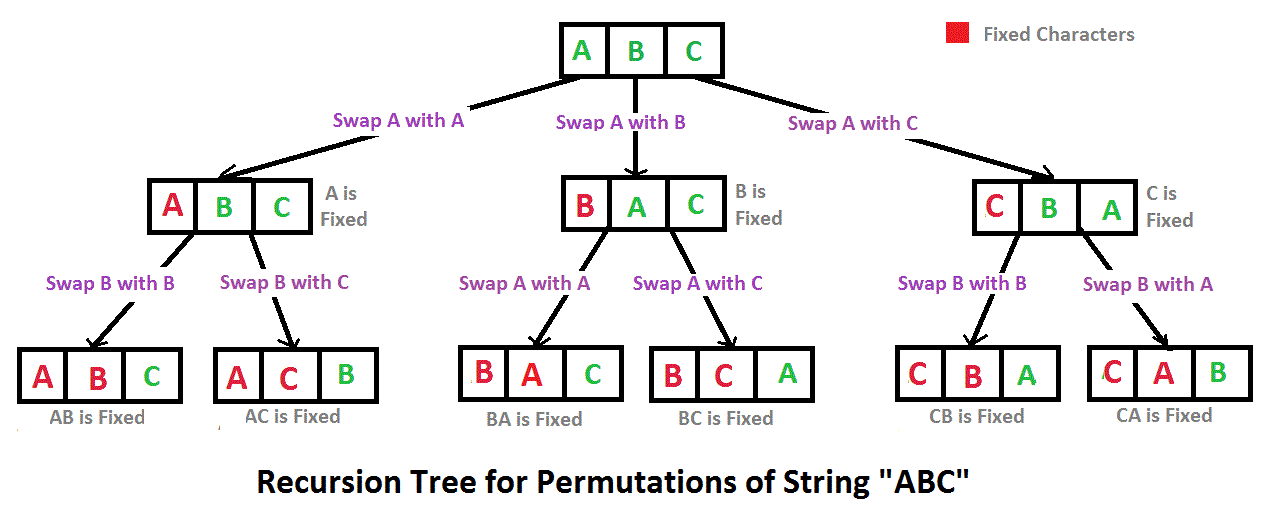# Print all possible permutations of a given string.

Permutation refers to the list of all possible ways in which a set or number of things can be arranged or ordered. The number of permutations possible depends on the number of elements in the list or number of characters in a string. For instance, if there are 'n' distinct characters in an array 'n!' permutations of the string are possible.

Below are the permutations of string XYZ.
XYZ XZY YXZ YZX ZYX ZXY

Here is a solution that is used as a basis for backtracking. Consider the input string to be 'ABC',## C/C++ implementation to print all permutations of a given string.

```// C program to print all permutations with duplicates allowed
#include <stdio.h>
#include <string.h>

/* Function to swap values at two pointers */
void swap(char *x, char *y)
{
char temp;
temp = *x;
*x = *y;
*y = temp;
}

/* Function to print permutations of string
This function takes three parameters:
1. String
2. Starting index of the string
3. Ending index of the string. */
void permute(char *a, int l, int r)
{
int i;
if (l == r)
printf("%s\n", a);
else
{
for (i = l; i <= r; i++)
{
swap((a+l), (a+i));
permute(a, l+1, r);
swap((a+l), (a+i)); //backtrack
}
}
}

/* Driver program to test above functions */
int main()
{
char str[] = "ABC";
int n = strlen(str);
permute(str, 0, n-1);
return 0;
}
```

## Java implementation to print all permutations of a given string.

```// Java program to print all permutations of a given string.
public class Permutation
{
public static void main(String[] args)
{
String str = "ABC";
int n = str.length();
Permutation permutation = new Permutation();
permutation.permute(str, 0, n-1);
}

private void permute(String str, int l, int r)
{
if (l == r)
System.out.println(str);
else
{
for (int i = l; i <= r; i++)
{
str = swap(str,l,i);
permute(str, l+1, r);
str = swap(str,l,i);
}
}
}

//Swap Characters at position

public String swap(String a, int i, int j)
{
char temp;
char[] charArray = a.toCharArray();
temp = charArray[i] ;
charArray[i] = charArray[j];
charArray[j] = temp;
return String.valueOf(charArray);
}

}

```

## Python implementation to print all permutations of a given string.

```# Python program to print all permutations with duplicates allowed

def toString(List):
return ''.join(List)

# Function to print permutations of string. This function takes three parameters:
# 1. String
# 2. Starting index of the string
# 3. Ending index of the string.
def permute(a, l, r):
if l==r:
print toString(a)
else:
for i in xrange(l,r+1):
a[l], a[i] = a[i], a[l]
permute(a, l+1, r)
a[l], a[i] = a[i], a[l] # backtrack

# Driver program to test the above function
string = "ABC"
n = len(string)
a = list(string)
permute(a, 0, n-1)
```

Output:

```ABC
ACB
BAC
BCA
CBA
CAB```

The above-implemented algorithms use backtracking algorithm paradigm.

The time complexity to print an element is computed to be O(n) whereas the complexity is seen to be O(n*n!) to compute all the permutations of a string with 'n' unique characters.

POST A NEW COMMENT

• Input (stdin)

Output (stdout)

Input (stdin)

Expected Output

Compiler Message

Input (stdin)

`2    3`

`5`
`5`
`5`